# 略微加速

## 略速 - 互联网笔记

2017-07-10 09:14:58 leiting (3217阅读)

## 什么是梯度下降和链式求导法则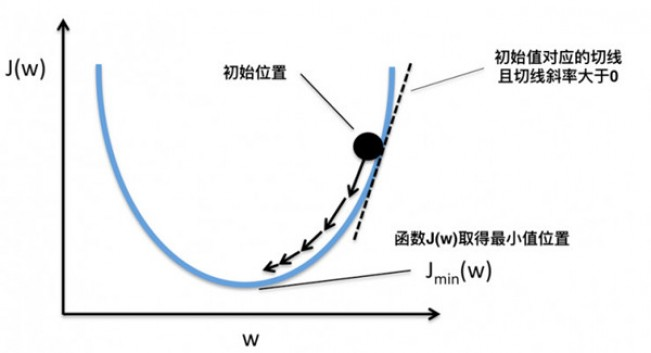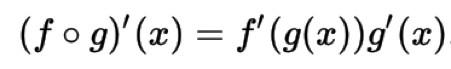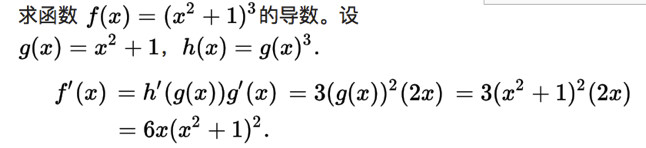## 神经网络的结构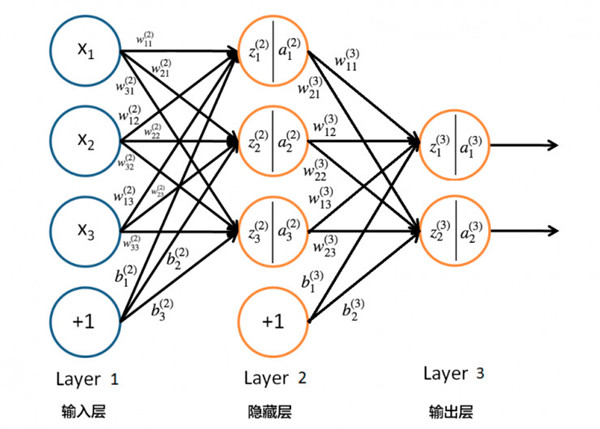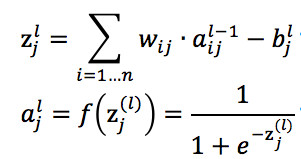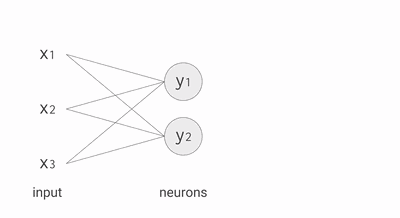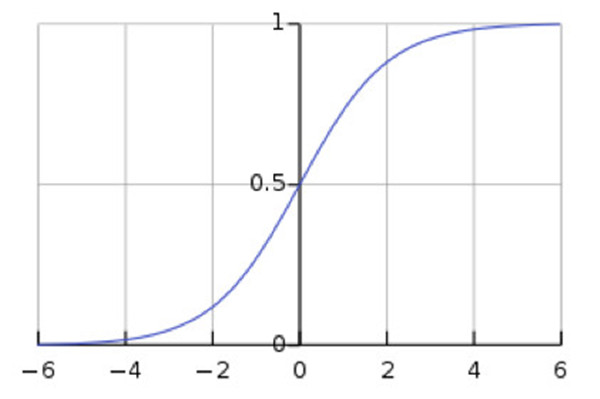## BP 算法执行的流程（前向传递和逆向更新）

● 权重的更新低于某个阈值的时候

● 预测的错误率低于某个阈值

● 达到预设一定的迭代次数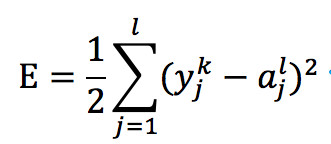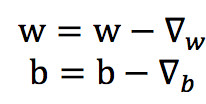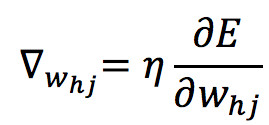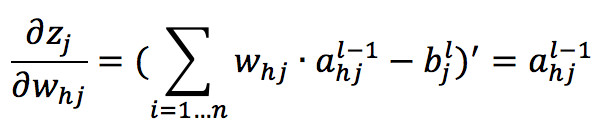Sigmoid 求导数的式子如下，从式子中可以发现其在计算机中实现也是非常的方便：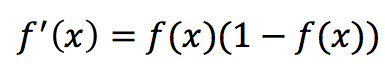Sigmoid 函数求导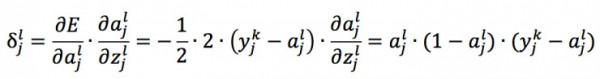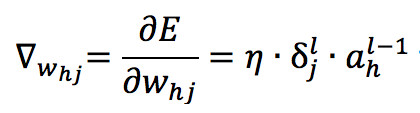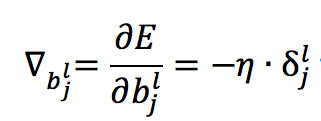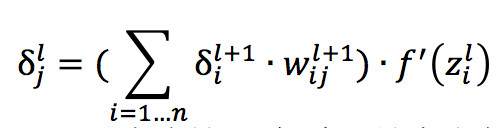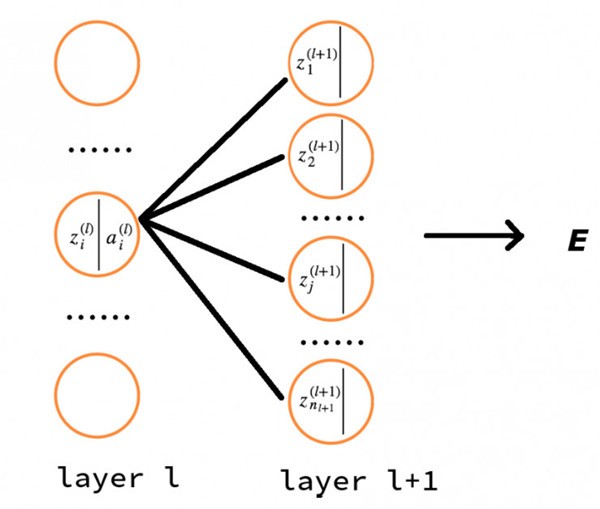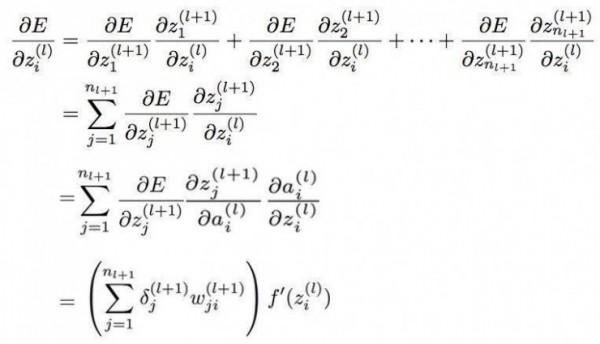## Python 源码解析

```def __init__(self, sizes):
"""
:param sizes: list类型，储存每层神经网络的神经元数目
譬如说：sizes = [2, 3, 2] 表示输入层有两个神经元、
隐藏层有3个神经元以及输出层有2个神经元
"""
# 有几层神经网络
self.num_layers = len(sizes)
self.sizes = sizes
# 除去输入层，随机产生每层中 y 个神经元的 biase 值（0 - 1）
self.biases = [np.random.randn(y, 1) for y in sizes[1:]]
# 随机产生每条连接线的 weight 值（0 - 1）
self.weights = [np.random.randn(y, x)
for x, y in zip(sizes[:-1], sizes[1:])]```

```def feedforward(self, a):
"""
前向传输计算每个神经元的值
:param a: 输入值
:return: 计算后每个神经元的值
"""
for b, w in zip(self.biases, self.weights):
# 加权求和以及加上 biase
a = sigmoid(np.dot(w, a)+b)
return a```

```def SGD(self, training_data, epochs, mini_batch_size, eta,
test_data=None):
"""
随机梯度下降
:param training_data: 输入的训练集
:param epochs: 迭代次数
:param mini_batch_size: 小样本数量
:param eta: 学习率
:param test_data: 测试数据集
"""
if test_data: n_test = len(test_data)
n = len(training_data)
for j in xrange(epochs):
# 搅乱训练集，让其排序顺序发生变化
random.shuffle(training_data)
# 按照小样本数量划分训练集
mini_batches = [
training_data[k:k+mini_batch_size]
for k in xrange(0, n, mini_batch_size)]
for mini_batch in mini_batches:
# 根据每个小样本来更新 w 和 b，代码在下一段
self.update_mini_batch(mini_batch, eta)
# 输出测试每轮结束后，神经网络的准确度
if test_data:
print "Epoch {0}: {1} / {2}".format(
j, self.evaluate(test_data), n_test)
else:
print "Epoch {0} complete".format(j)```

```def update_mini_batch(self, mini_batch, eta):
"""
更新 w 和 b 的值
:param mini_batch: 一部分的样本
:param eta: 学习率
"""
# 根据 biases 和 weights 的行列数创建对应的全部元素值为 0 的空矩阵
nabla_b = [np.zeros(b.shape) for b in self.biases]
nabla_w = [np.zeros(w.shape) for w in self.weights]
for x, y in mini_batch:
# 根据样本中的每一个输入 x 的其输出 y，计算 w 和 b 的偏导数
delta_nabla_b, delta_nabla_w = self.backprop(x, y)
# 累加储存偏导值 delta_nabla_b 和 delta_nabla_w
nabla_b = [nb+dnb for nb, dnb in zip(nabla_b, delta_nabla_b)]
nabla_w = [nw+dnw for nw, dnw in zip(nabla_w, delta_nabla_w)]
# 更新根据累加的偏导值更新 w 和 b，这里因为用了小样本，
# 所以 eta 要除于小样本的长度
self.weights = [w-(eta/len(mini_batch))*nw
for w, nw in zip(self.weights, nabla_w)]
self.biases = [b-(eta/len(mini_batch))*nb
for b, nb in zip(self.biases, nabla_b)]```

```def backprop(self, x, y):
"""
:param x:
:param y:
:return:
"""
nabla_b = [np.zeros(b.shape) for b in self.biases]
nabla_w = [np.zeros(w.shape) for w in self.weights]
# 前向传输
activation = x
# 储存每层的神经元的值的矩阵，下面循环会 append 每层的神经元的值
activations = [x]
# 储存每个未经过 sigmoid 计算的神经元的值
zs = []
for b, w in zip(self.biases, self.weights):
z = np.dot(w, activation)+b
zs.append(z)
activation = sigmoid(z)
activations.append(activation)
# 求 δ 的值
delta = self.cost_derivative(activations[-1], y) * \
sigmoid_prime(zs[-1])
nabla_b[-1] = delta
# 乘于前一层的输出值
nabla_w[-1] = np.dot(delta, activations[-2].transpose())
for l in xrange(2, self.num_layers):
# 从倒数第 **l** 层开始更新，**-l** 是 python 中特有的语法表示从倒数第 l 层开始计算
# 下面这里利用 **l+1** 层的 δ 值来计算 **l** 的 δ 值
z = zs[-l]
sp = sigmoid_prime(z)
delta = np.dot(self.weights[-l+1].transpose(), delta) * sp
nabla_b[-l] = delta
nabla_w[-l] = np.dot(delta, activations[-l-1].transpose())
return (nabla_b, nabla_w)```

```def evaluate(self, test_data):
# 获得预测结果
test_results = [(np.argmax(self.feedforward(x)), y)
for (x, y) in test_data]
# 返回正确识别的个数
return sum(int(x == y) for (x, y) in test_results)```

```import mnist_loader
import network

net = network.Network([784, 30, 10])
net.SGD(training_data, 30, 10, 3.0, test_data = test_data)
# 输出结果
# Epoch 0: 9038 / 10000
# Epoch 1: 9178 / 10000
# Epoch 2: 9231 / 10000
# ...
# Epoch 27: 9483 / 10000
# Epoch 28: 9485 / 10000
# Epoch 29: 9477 / 10000```

## TODO

• 如何选择超参数的值，譬如说神经网络的层数和每层的神经元数量以及学习率；

• 既然对初始化权重敏感，那该如何避免和修正；

• Sigmoid 激活函数在深度神经网络中会面临梯度消失问题该如何解决；

• 避免 Overfitting 的 L1 和 L2正则化是什么。

## 参考

 周志华 机器学习

 斯坦福大学机器学习在线课程

 Parallel Distributed Processing (1986, by David E. Rumelhart, James L. McClelland), Chapter 8 Learning Internal Representations by Error Propagation

 How the backpropagation algorithm works

 Backpropagation Algorithm